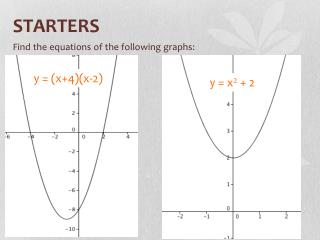# STARTERS - PowerPoint PPT PresentationDownload PresentationSTARTERS

STARTERSDownload Presentation## STARTERS

- - - - - - - - - - - - - - - - - - - - - - - - - - - E N D - - - - - - - - - - - - - - - - - - - - - - - - - - -
##### Presentation Transcript

1. STARTERS Find the equations of the following graphs: y = (x+4)(x-2) y = x2 + 2

2. Note 7: Cubics - Basic y = x3 This “central point” is called a “point of inflexion”

3. Any functions of the basic cubic are treated the same as the shifts in the parabola. Examples: Graph y = x3 + 4 y = x3 + 4 y-intercept is 4

4. Examples: Graph y = (x – 5)3 + 2 Vertex (5, 2) y = (x - 5)3 + 2

5. Graph the following cubics y = x3 + 1 y = (x – 2)3 y = -x3y = (x + 1)3 – 2 y = x3– 3 y = (x – 3)3